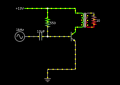Single Transistor Amplifier using NTE 396 - wierd beta and heat behaviour

tomerp

Joined Jul 27, 2018
1
hi All
Im experimenting with single transistor amplifier. I am using NTE 396 in the following circuit:here are some notes on the circuit:

- The 10 ohm resistor on the far right represents what would be a speaker
- The transistor is NTE 396 which is a small power transistor
- The transformer primary resistance in pure DC is 26ohm
- Im feeding it 19Volts since i want high power output
- note that my base bias resistor is 590Ohm (not 550 as it says). so i calculate the current in pure DC to be (19-0.7)/590=31ma
- the DC voltage i measure is 4.75 volts on the primary of the transformer. so it works out to about 4.75/26=180ma current. This gives a beta of only 180/31=5

here are my questions:
-Why is the measured current (and voltage) on the primary of the transfomer, in pure DC, not much higher? how come the beta is only 5?
- Assuming the transistor has beta of at least 50 this works out to a max of 1.55Amps (with 31ma base current). With 19Volts, i would expect then expect around 18 volts dropping on the transformer and current to be like 18/26=0.7A. so why is it only 180ma?
- Also (i know its bad) the transistor has turned brown (it still works but has browned up). any ideas why? i would expect it to be able to handle 180ma wihtout browning

Thanks

phranzdan

Joined Aug 4, 2017
40
There are a lot of questions relative to the conditions you stated. First of all, the 4.75 volts you mention on the primary. I assume you are measuring this directly across the primary. If that is the case, then the current in the collector circuit of the transistor is 180 ma. Since the supply is 19 volts, that leaves about 14 volts collector to emitter. The power dissipated in the transistor under these conditions would be about 2.5 watts. The junction to ambient thermal resistance of the NE396 is 175 deg c/ watt giving a junction temperature of 2.5 x 175 or 437 deg. c assuming you are not using any heat sinking. That is enough to turn the transistor brown. Not certain what you are trying to accomplish with this circuit so I can't offer any help.

crutschow

Joined Mar 14, 2008
28,458
i would expect it to be able to handle 180ma wihtout browning
Then you obviously didn't calculate its power dissipation.
The maximum power dissipated by the transistor can be as high as 3.5W with a 19v supply and a 26Ω transformer.
The high transistor dissipation shows why Class A audio amps are not typically used to power a speaker.

You beta is low because the transistor was zapped by overheating (as the brown color indicates).